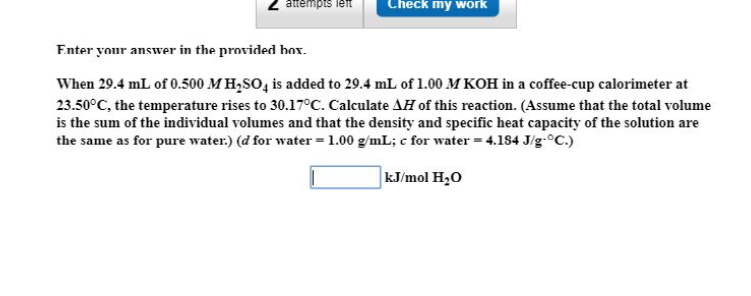# When 29.4 ml. of 0.500 M H2SO4 is added to 29.4 ml. of 1.00 M KOH in a coffee-cup calorimeter at 23.50C, the temperature rises to 30.17C. Calculate [d]H of this reaction. (Assume that the total volume is the sum of the individual volumes and that the density and speciﬁc heat capacity of the solution are the same as for pure water.) [d for water = 1.00 g/ml; c for water = 4.184 J/g C)